Wednesday , December 6 2023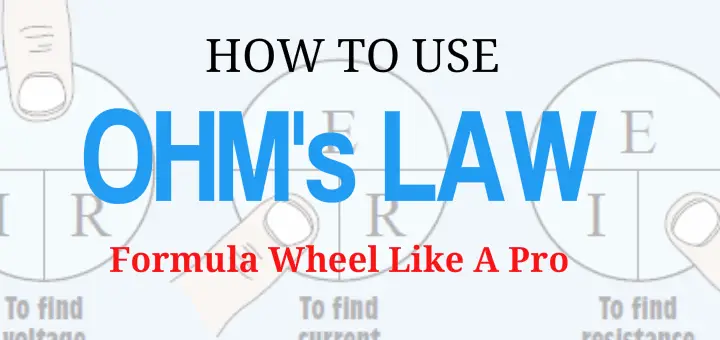# Ohms Law Power Wheel: Understanding the Electrical Formula Wheel

Last updated on July 30th, 2023.

As an electrician, if there’s one thing that you need to have a solid understanding of – it’s Ohm’s Law. This simple formula allows you to explore the relationship between three electrical variables: voltage, current, and resistance.

The good thing is that it’s not rocket science. If you know how to multiply and divide this will be a walk in the park. An easy way to understand Ohms law is through the Ohms Law Wheel.

## How To Use The Ohm’s Law Formula Wheel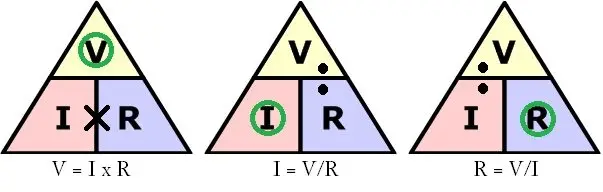I know that you’re thinking, “That’s a triangle.” Don’t worry about that, just pay attention. So you need to find out how many amps a circuit will draw and you can’t put an ammeter on it. What do you do?

Simple, divide the VOLTAGE by the RESISTANCE of the circuit. How do you know this? Because of the Ohm’s Law formula wheel.

In the formula wheel you’ll see three letters – each one represent a value.

### R= RESISTANCE (ohms)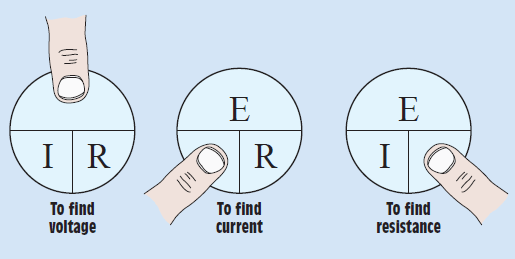So, if you need to find voltage, current, or resistance, simply place your finger over what you’re trying to find and the formula wheel will do the rest.

The Ohm’s law formula wheel is represented mathematically in three simple equations.

I (current) x R (resistance) = E (voltage)

E (voltage) ÷ R (resistance) = I (current)

E (voltage) ÷ I (current) = R (resistance)

## Ohm’s Law Example Problems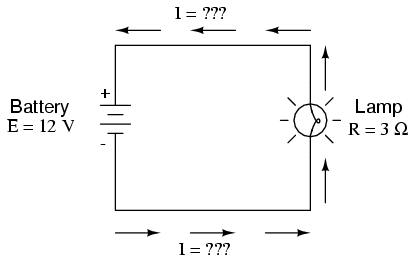Find the resistance of the circuit. By looking at this circuit we know the values of two components: voltage (12v) and resistance (3 ohms). How do we find current?

We plug our known values into our formula wheel and work the equation.

12 volts ÷ 3 ohms = 4 amps

It’s really that simple. Here, we’ll try another. Find the resistance in the circuit with the values below:

Voltage = 120v

Current = 17 amps

Now plug the known values into our formula wheel and work the equation.

120 volts ÷ 17 amps =  7.05 ohms

I told you it was simple. Easier than installing a whole house surge protector er?

(okay maybe not that easy. But definitely easier than installing a refrigerator surge protector (you just plug the damn thing in)!)

## Principle’s of Ohm’s Law – Proportional and Inversely Proportional

There is a rule about ohm’s law that you need to be familiar with.

that the electrical current (I) flowing in an circuit is proportional to the voltage (V) and inversely proportional to the resistance (R).

This means that if the voltage is increased then the current will increase as long as the resistance doesn’t change. If the resistance is increased and voltage remains the same, then the current will decrease.

### Increasing Resistance

120 volts ÷ 5 ohms = 60 amps

120 volts ÷ 10 ohms = 12 amps

120 volts ÷ 20 ohms = 6 amps

Therefore, if the voltage is increased, the current will increase provided the resistance of the circuit does not change.

### Increasing Voltage

120 volts ÷ 25 ohms = 4.8 amps

240 volts ÷ 25 ohms = 9.6 amps

480 volts ÷ 25 ohms = 19.2 amps

As you can see when we increase the voltage and leave the resistance the same, the current increased (directly proportional to the voltage).

## Ohm’s Law PIE Formula Chart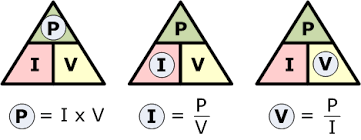The PIE chart is similar to the voltage, current, and resistance formula wheel. Power is measured in watts and is defined as:

the rate at which work is done when one ampere (A) of current flows through an electrical potential difference of one volt (V)

## Formula Wheel with Ohm’s Law and PIE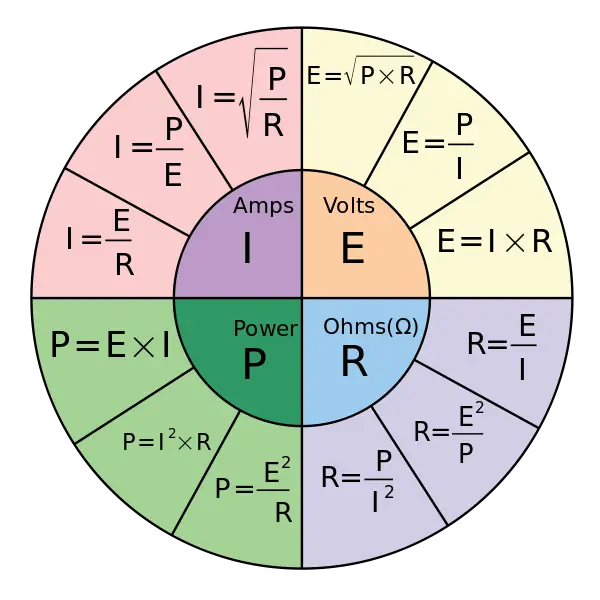Now we’re getting somewhere. This formula wheel is a combination of both Ohm’s Law and the PIE formula.

It looks more complicated but in reality, it’s easy to use (you may need a calculator), and it works the same way as the previous charts.

The formula wheel is divided into four sections, each section has three formulas. If you need to find volts then you would use the E section, current – the I section, resistance – the R section, and power – the P section.

When using the formula wheel you need to follow these steps:

1. Know what you’re trying to solve for: current (I), voltage (E), resistance (R), or power (P).
2. What values you already know (you need two): current (I), voltage (E), resistance (R), or power (P)
3. Find the section of the formula wheel that your values plug into.
4. Solve the equation

When making calculations you have to use compatible values. What I mean is kilohms should be converted to ohms, milliamperes should be converted to amperes.

Whether you’re an electrician apprenticeship or Journeyman electrician, learning Ohm’s law is an essential part of an electricians role.